Pay

## Interface Call Sequence Diagram

9
0
0
publish time: 2021-03-09Kiraaaa

This template is an interface call sequence diagram about payment functions, a detailed description of the call interface. Sequence diagrams are commonly used by developers to depict interactions between objects in a use case (actions that show interactions between an actor and a system to achieve a goal). A brief introduction to the call of the interface, let us take a look.

See More Related TemplatesThis is an example of a UML diagram about adding events to the calendar.
UML Diagram - Add event to calendar
15
1
0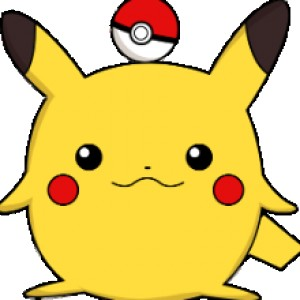easy diagrams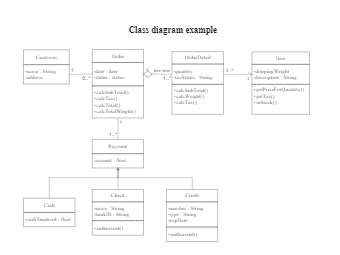This is an example of a uml class diagram.
Class diagram example
151
4
3easy diagrams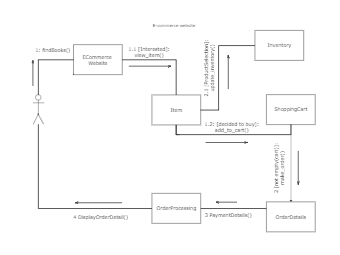This is an example of a UML collaboration diagram.
ecommerce website - UML diagram
9
1
0easy diagrams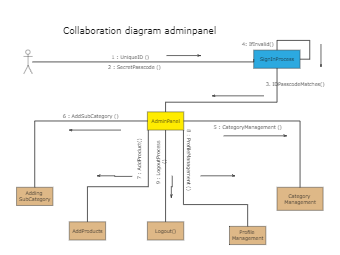This is an example of a UML collaboration diagram about adminpanel.
Collaboration diagram adminpanel
6
1
0easy diagrams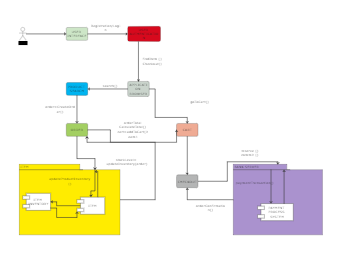This is an example of a UML communication diagram.
Communication diagram example
8
1
0easy diagrams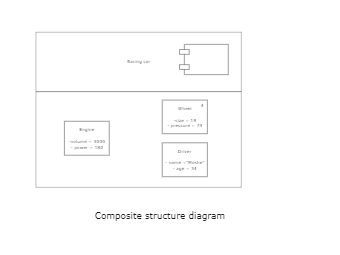This is an example of a composite structure diagram.
Composite structure diagram
3
0
0easy diagrams